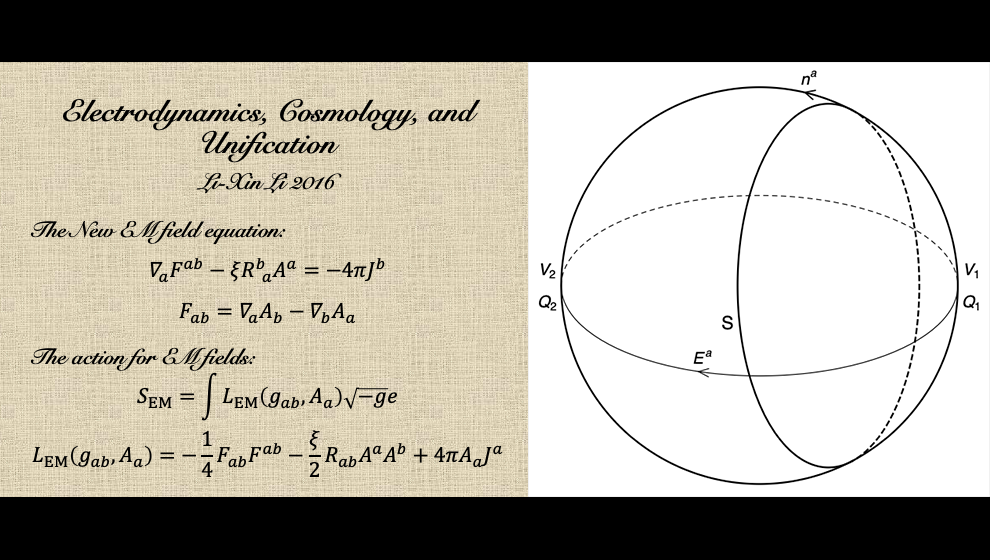## Li-Xin LiMaxwell's equations cannot describe a homogeneous and isotropic universe with a uniformly distributed net charge, because the electromagnetic field tensor in such a universe must be vanishing everywhere. For a closed universe with a nonzero net charge, Maxwell's equations always fail regardless of the spacetime symmetry and the charge distribution. The two paradoxes indicate that Maxwell's equations need be modified to be applicable to the universe as a whole.

In a paper published in General Relativity and Gravitation in 2016, I considered two types of modified Maxwell equations, both can address the paradoxes. One is the Proca-type equation which contains a photon-mass term. This type of electromagnetic field equations can naturally arise from spontaneous symmetry breaking and the Higgs mechanism in quantum field theory, where photons acquire a mass by eating massless Goldstone bosons. However, photons loose their mass again when the symmetry is restored, and the paradoxes reappear. The other type of modified Maxwell equations, which are more attractive in my opinions, contain a term with the potential vector of the electromagnetic field coupled to the curvature tensor of the spacetime (the field equation in the above figure):

(1)                 ∇aFab - ξRbaAa = -4πJb ,

where Fab is the antisymmetric electromagnetic field tensor defined by the potential vector Aa, Rab is the Ricci curvature tensor of the spacetime, and Ja is the electric current-density vector. This type of electromagnetic field equations do not introduce a new dimensional parameter and return to Maxwell's equations in a flat or Ricci-flat spacetime. The curvature-coupled term ξRbaAa can naturally arise from the ambiguity in extending Maxwell's equations from a flat spacetime to a curved spacetime through the "minimal substitution" rule. [For details see L.-X. Li, Gen. Relativ. Gravit. 48, 28 (2016).]

In a subsequent paper published in Frontiers of Physics in 2016, I presented a new unified theory (NUT) of electromagnetic and gravitational interactions. By considering a four-dimensional spacetime as a hypersurface embedded in a five-dimensional bulk spacetime, I derived the complete set of field equations in the four-dimensional spacetime from the five-dimensional Einstein field equation. Besides the Einstein field equation in the four-dimensional spacetime, an electromagnetic field equation is obtained, which just has the form of the new electromagnetic field equation (1) but with ξ = -2. The NUT is physically different from Kaluza-Klein theory and its variants in which the Einstein-Maxwell equation is derived.

In the four-dimensional Einstein field equation derived in the NUT, the source term includes the stress-energy tensor of electromagnetic fields as well as the stress-energy tensor of other unidentified matter. Under certain conditions the unidentified matter can be interpreted as a cosmological constant in the four-dimensional spacetime. The new electromagnetic field equation (1) and the NUT described above can be tested in an environment with a high mass density, e.g., inside a neutron star or a white dwarf, and in the early epoch of the universe. [For details see L.-X. Li, Front. Phys. 11, 110402 (2016).]Back to Li-Xin Li's Web Page